# Integrator Amplifier

## Working principle

An integrator produces an output voltage, which is proportional to the integral of the input voltage: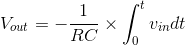### Formula derivation

Because of virtual ground and infinite impedance of the op-amp’s input terminals, all of the input current flows through R and C: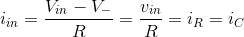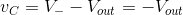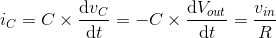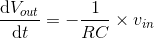An application for this circuit could be integrating water flow and measuring the total quantity of water that has passed by the flowmeter.

### Experiment

Set the AC voltage source’s amplitude and frequency to 0 and the DC voltage source’s value to 1 V. Observe the output. If a fixed voltage is applied to the input of an integrator, the output voltage will be a ramp with a constant slope of the negative input voltage multiplied by a factor of 1/RC.